## Saturday, March 11, 2006

### scribe notes for march 10th

On Friday, Mr. Reece gave us a pop quiz to test us on some of the things we knew about angels. Some of the types of questions that were on the quiz were:

e.g. desribe the measurment as an angle.
a) 145 degrees = that measurment equals an obtuse angle because the measurment is greater then 90 degrees and less than 180 degrees.

e.g. Give an acurate diagram of the angle that is written.
a) acute angle: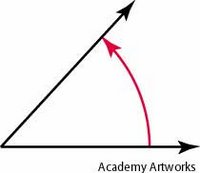This would be the correct type of diagram to draw because it is less then 90 degrees but it is greater than 0 degrees.

The quiz did not take that long and it was out of 10 marks.

After we reviewed Geometry terms on the smart board and we did a search for different lines in Winnipeg. An example of a line in Winnipeg would be the Pembina Highway. It would be an example of a line in Winnipeg because it would keep on going.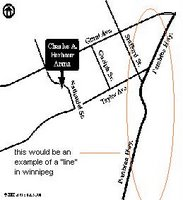We learned two new terms in math. They are:

Intersectings lines: two lines that cross each other in exactly one place.
e.g.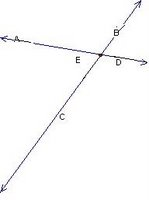Perpendicular Lines: Two intersecting lines that cross at 90 degrees to one another.
e.g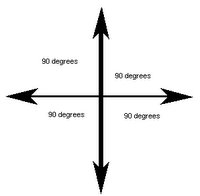We had some homework due on Friday it was the three point approach. Try to finish it if you haven't because it will help you study for tests.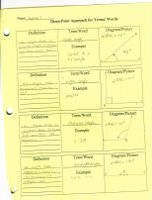This is what it may look like.

Homework: questions 1 to 2 and 5-10 on page 265. Due for monday and there is homework on Mr. Reece's blog go check it out math blogorama

The next scribe will be: Amy=)

### Homework

Hi everybody!

There is homework posted on Mr. Reece's Math Blogorama due Thursday, March 16.

Remember to do the homework and get the marks.

## Tuesday, March 07, 2006

### March 7/2006 scribe notes

I, Ardia Sarao, am the scribe for today. This is what we learned:

we learned about writing angles, here are pictures and explanations of what we learned today: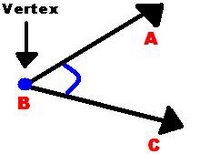The angle can be written as angle ABC or angle CBA

Then we had to draw these three angles: Obtuse Acute Reflex
They are suppost to look like this: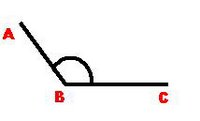The Obtuse angle is an angle that is more then 90° and less then 180°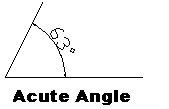An acute angle is an angle that is between 0 and 90°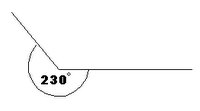And finally the Reflex angle is one which is more than 180° but less than 360°

Then we had to draw 2 more angles and they were: complimentary supplimentary

Complimentary angles are two angles that measures add up to 90°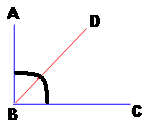Supplementary angles
are two angles that me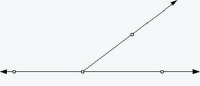asures add up to 180°

Then we had to do these questions that we had to look at these pictures on lines and angles and had to find a 3 names that suits it.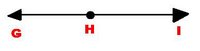1) straight angle, angle GHI , 180°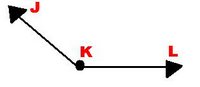2)Reflex angle, obtuce angle, angle JKL

The HOMEWORK for today was to finish the 3 point approach definitions of your angles and stuff for Firday March 10/2006 and BRING YOUR TEXT BOOK!!!

Next scribe:
is Jaymie!!!!=P

## Monday, March 06, 2006

### Monday March 6; Scribe

During Class:
During class today, we spent a few minutes reviewing the five angles we learned on Thursday. If you were not here Thursday here are the types of angles: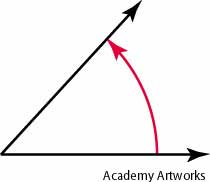The Acute Angle:
Any angle between
0-90 degrees.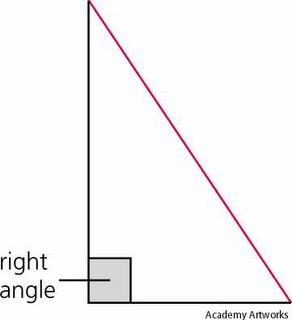Right Angle:

An angle that IS EXACTLY 90 degrees.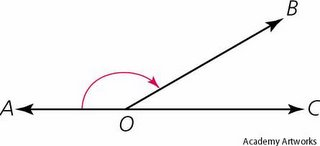Obtuse Angle:
Any angle GREATER THAN 90 degrees, but LESS THAN 180 degrees.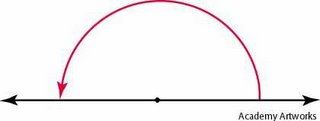Straight Angle:
Any angle that IS EXACTLY 180 degrees.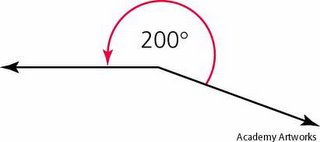Reflex Angle:

Any angle that is GREATER THAN 180 degrees, but LESS THAN 360 degrees.

After that we spent our time writing down geometric terms. Here are the terms:

Point:
A geometric element that indicates position, but has no size.(You cannot measure a point.)

Example: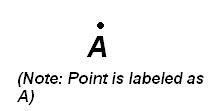Line:

Are a set of points in a straight line. They extend forever in both directions.

Example: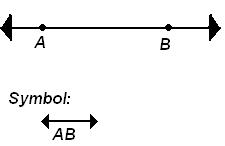Ray:
A set of points that form a straight line. A Ray starts at a point and extends in ONE direction forever.

Example: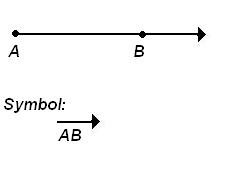Line Segment:
Apart of a line with two end points.

Example: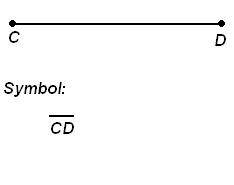After writing down those diagrams and definitions, we were asked to see what were the different types of symbols there were in this diagram: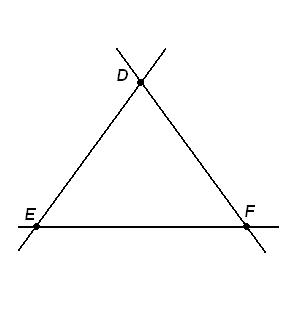We have 3 lines, 3 lines segments and 6 rays:
Lines: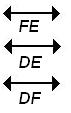Line Segments: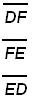Rays: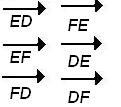HOMEWORK:

Page 262; Questions 1-5, 7-16. DO NOT LOOK AT THE BACK OF THE BOOK LIKE SOME PEOPLE! YOU WILL LEARN NOTHING!!

Next Scribe:
If you can, Ardia, will you please be the next scribe?